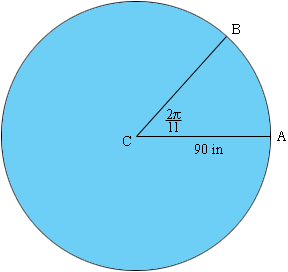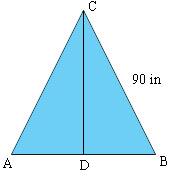Quandaries and Queries My name is Bob and I have BA degree and two Associate degrees, but been out of school a long time.  Here is the problem.  I have a 15' circular above ground pool.  Around the perimeter of the pool are eleven (11) sections of railing.  Each rail has 5 slots at each end for pins.  I have calculated that the length of the arc under the railing to be 51.4".  what I am trying to determine is the distance between the end points of the arc so that I can figure out which slot to use in the rails without going round and round the pool moving and removing the rails until they finally fit.  Been there, done that, no fun.   Thanks, Bob Hi Bob, I sketched a diagram of your pool and one of the eleven segments that are defined by the sections of railing.I measured the angle in radians since the length a of the arc AB is given by a = rtheta where r is the radius and theta is the angle measured in radians. For your pool I got a = 902/11 = 51.4 inches as you did also. To find the distance between A and B I looked at the triangle ABC and let D be the midpoint of AB.The measure of angle BCD is/11 and sin(BCD) = |DB|/|BC| and hence |AB| = 2|DB| = 2|BC|sin(/11) = 50.71 inches Penny Go to Math Central